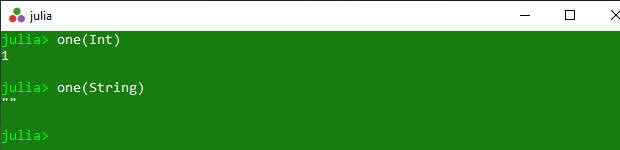Related Articles
Getting multiplicative identity in Julia – one() Method
• Last Updated : 01 Apr, 2020

The `one()` is an inbuilt function in julia which is used to return a multiplicative identity of the specified value x, where x is a value such that one(x)*x == x*one(x) == x.

Syntax:
one(x)
or
one(T::type)

Parameters:

• : Specified value.
• one(T::type): Specified number type.

Returns: It returns a multiplicative identity of the specified value x, where x is a value such that one(x)*x == x*one(x) == x.

Example 1:

 `# Julia program to illustrate ``# the use of one() method``  ` `# Getting return a multiplicative ``# identity of the specified value x``println(one(``0``))``println(one(``1``))``println(one(``5``))``println(one(``5.9``))`

Output:

```1
1
1
1.0```

Example 2:

 `# Julia program to illustrate ``# the use of one() method``  ` `# Getting return a multiplicative ``# identity of the specified number type``println(one(``Int``))``println(one(String))`

Output:Attention reader! Don’t stop learning now. Get hold of all the important DSA concepts with the DSA Self Paced Course at a student-friendly price and become industry ready.

My Personal Notes arrow_drop_up